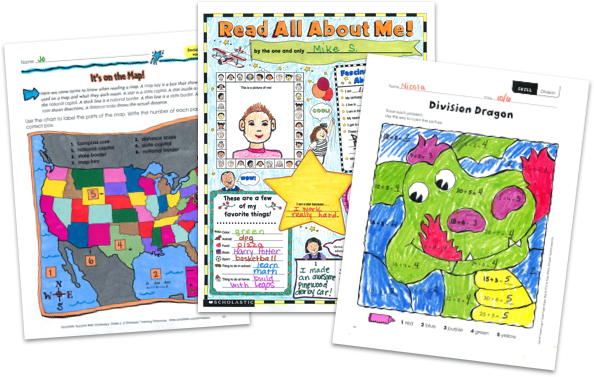MY FILE CABINET# Math Lesson Plans & Worksheets From Scholastic TeachablesScholastic Teachables (formerly Scholastic Printables) has more than 1,500 math lesson plans and teaching ideas for grades Pre-K through 8 that span more than 25 different math concepts and skills! Sign up today and get instant online access to math lesson plans, as well as worksheets, practice pages, and more to cover all grades and skills.

Scholastic Teachables (formerly Scholastic Printables) has more than 1,500 math lesson plans and teaching ideas for grades Pre-K through 8 that span more than 25 different math concepts and skills! Sign up today and get instant online access to math lesson plans, as well as worksheets, practice pages, and more to cover all grades and skills.

## Scholastic's Math Lesson Plans Cover:

• Algebra
• Charts and Graphs
• Counting and Numbers
• Data Analysis
• Early Math
• Estimation
• Fractions and Decimals
• Geometry
• Logic and Problem Solving
• Math Fluency and Intervention
• Money Math
• Multiplication and Division
• Number Sense
• Order of Operations
• Patterns and Symmetry
• Place Value
• Properties
• Ratio, Proportion, and Scale
• Real-World Math
• Sequencing
• Time and Measurement
• Word Problems
• And More!

## Sample Math Lesson Plans

More than 1,500 printable math lesson plans, teaching ideas, and strategies span more than 25 math topics, concepts, and skills from Pre-K through grade 8. Scholastic publishes new printable math lesson plans and worksheets each month to keep your courses fresh, exciting, and up-to-date, and you can access all of our resources from any device, whether at school or at home. Spend more time focusing on your courses and less time on preparing materials—simply find it, print it, teach it!

Here's a sample of lesson plans for you to try in your class—FREE with a 30-day trial or subscription.

## Scholastic's Math Lesson Plans Cover:

• Algebra
• Charts and Graphs
• Counting and Numbers
• Data Analysis
• Early Math
• Estimation
• Fractions and Decimals
• Geometry
• Logic and Problem Solving
• Math Fluency and Intervention
• Money Math
• Multiplication and Division
• Number Sense
• Order of Operations
• Patterns and Symmetry
• Place Value
• Properties
• Ratio, Proportion, and Scale
• Real-World Math
• Sequencing
• Time and Measurement
• Word Problems
• And More!
• Algebra
• Charts and Graphs
• Counting and Numbers
• Data Analysis
• Early Math
• Estimation
• Fractions and Decimals
• Geometry
• Logic and Problem Solving
• Math Fluency and Intervention
• Money Math
• Multiplication and Division
• Number Sense
• Order of Operations
• Patterns and Symmetry
• Place Value
• Properties
• Ratio, Proportion, and Scale
• Real-World Math
• Sequencing
• Time and Measurement
• Word Problems
• And More!

## Sample Math Lesson Plans

More than 1,500 printable math lesson plans, teaching ideas, and strategies span more than 25 math topics, concepts, and skills from Pre-K through grade 8. Scholastic publishes new printable math lesson plans and worksheets each month to keep your courses fresh, exciting, and up-to-date, and you can access all of our resources from any device, whether at school or at home. Spend more time focusing on your courses and less time on preparing materials—simply find it, print it, teach it!

Here's a sample of lesson plans for you to try in your class—FREE with a 30-day trial or subscription.## Additional Alphabet Worksheets (Subscription Required)

Try FREE for 30 days!

If you are not 100% satisfied cancel during the FREE trial and owe nothing. If you are not 100% satisfied cancel during the FREE trial and owe nothing.